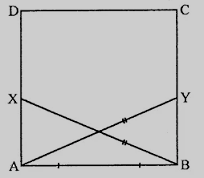# $ABCD$ is a square, $X$ and $Y$ are points on sides $AD$ and $BC$ respectively such that $AY = BX$. Prove that $BY = AX$ and $\angle BAY = \angle ABX$.

Given:

$ABCD$ is a square, $X$ and $Y$ are points on sides $AD$ and $BC$ respectively such that $AY = BX$.

To do:

We have to prove that $BY = AX$ and $\angle BAY = \angle ABX$.

Solution:In $\triangle BAX$ and $\triangle ABY$,

$AB =AB$           (Common)

$BX = AY$

Therefore, by RHS axiom,

$\triangle BAX \cong \triangle ABY$

This implies,

$AX = BY$                   (CPCT)

$\angle ABX = \angle BAY$             (CPCT)

Hence, $BY = AX$ and $\angle BAY = \angle ABX$.